# Law of Cosines Calculator

Use our Law of Cosines calculator to find any angle or side of a triangle given other known properties.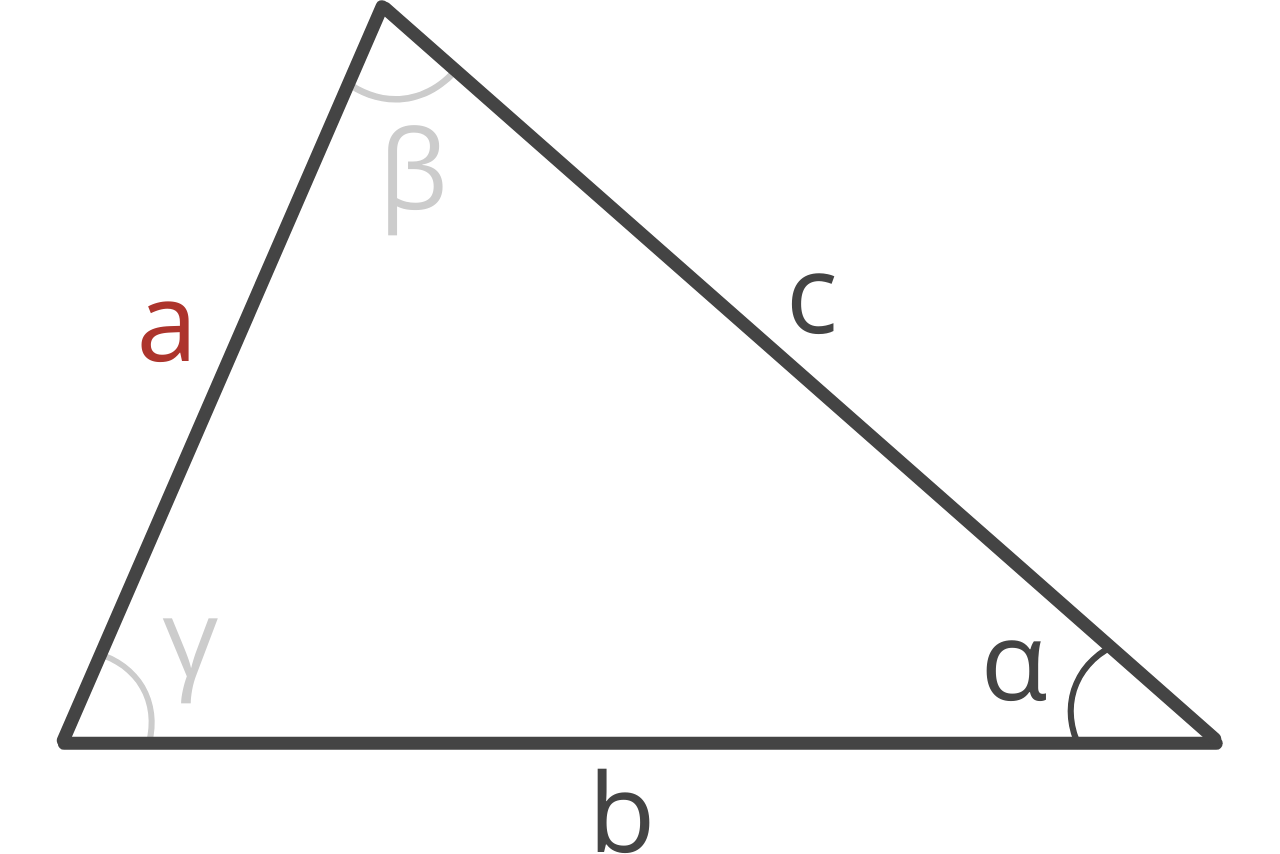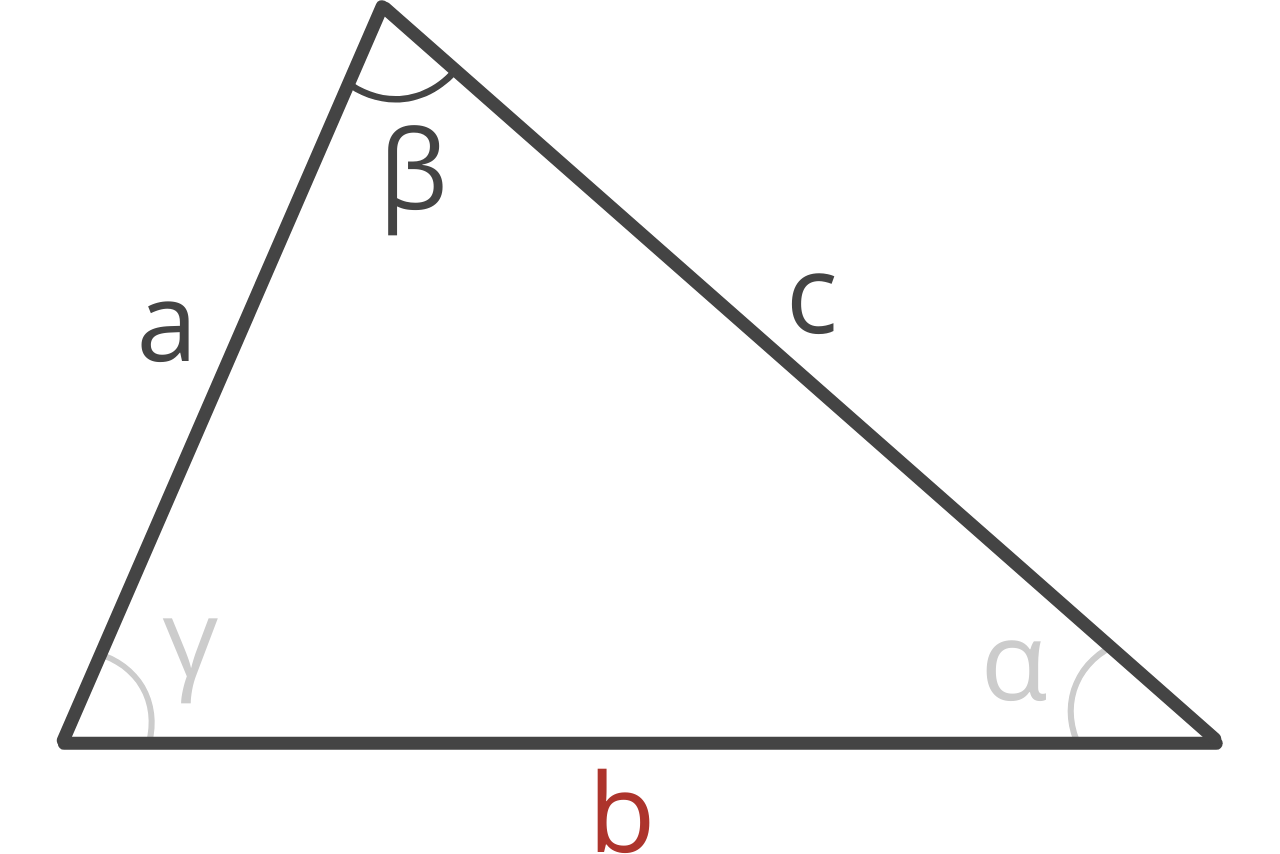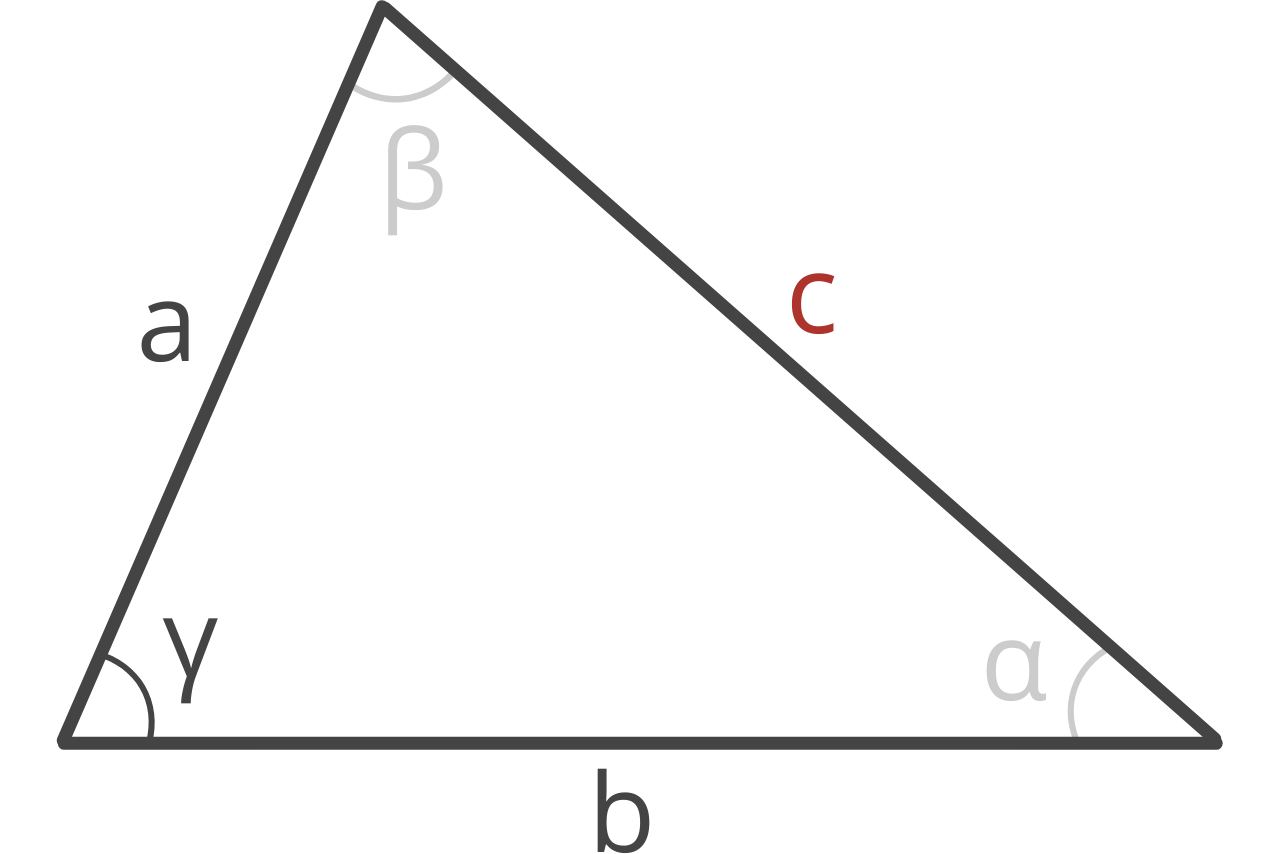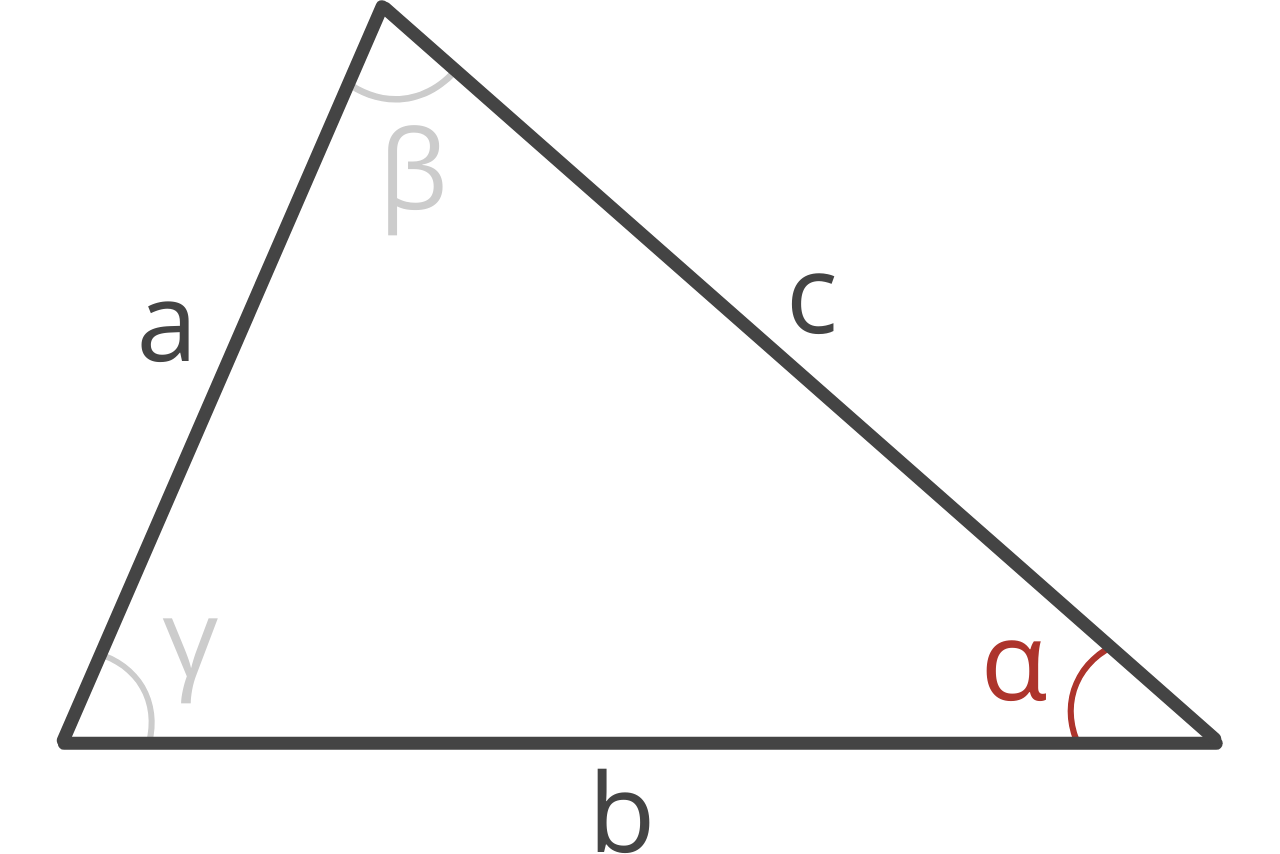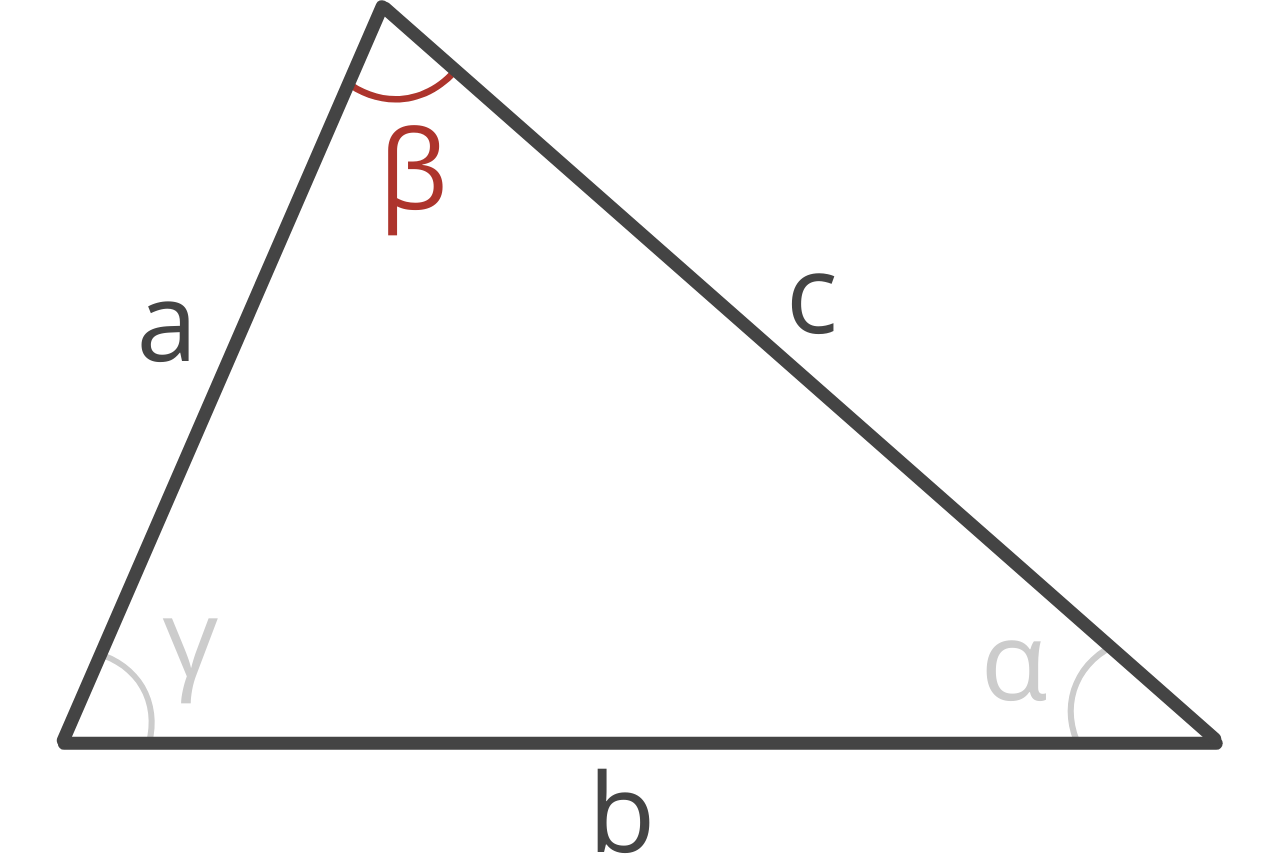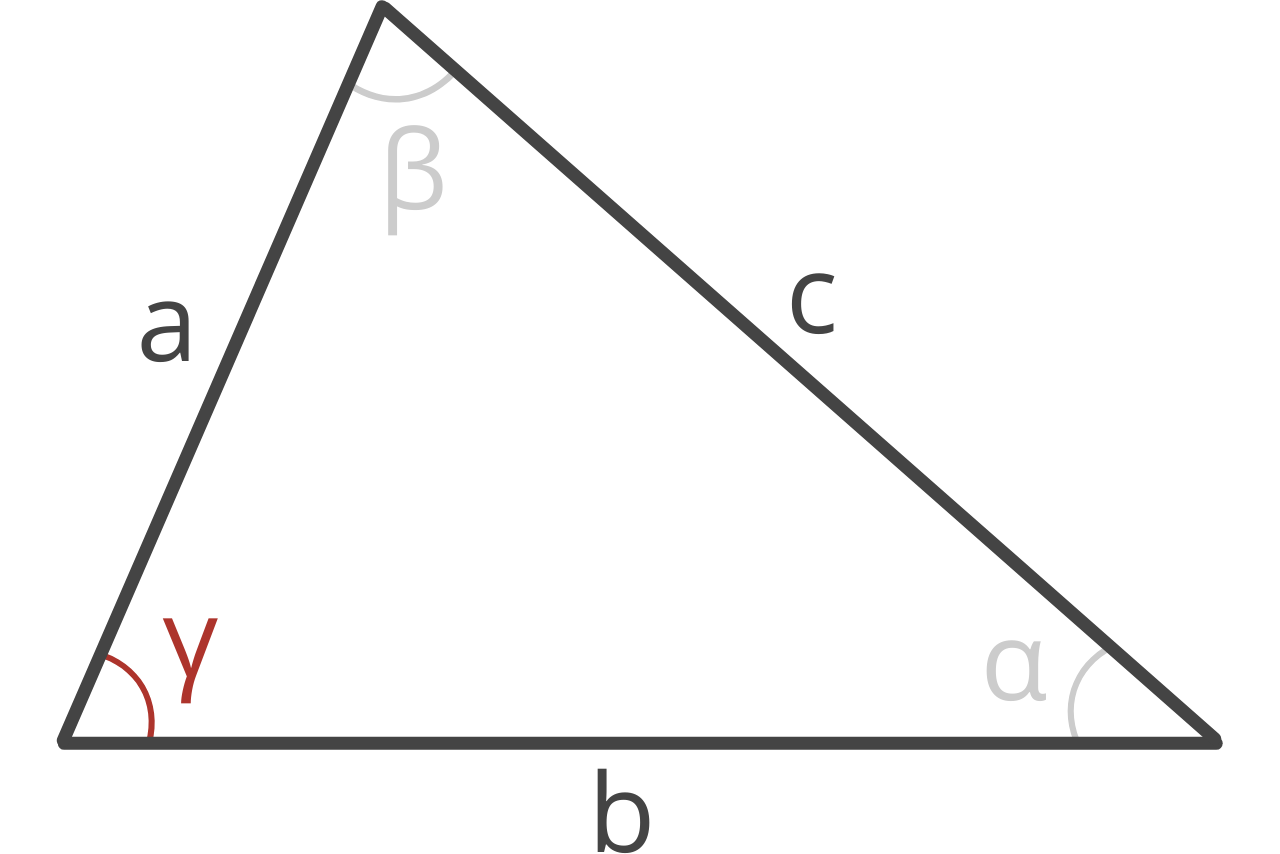## Result:

a = 23.0876

### Sides

 a = 23.0876 25 15

### Angles

α = 65 1.134464 Result Unit ° rad 78.9261 1.3775 Result Unit ° rad 36.0739 0.62961 Result Unit ° rad

### Steps to Solve

a² = b² + c² - 2bc cos(α)

a = b² + c² - 2bc cos(α)

a = 25² + 15² - 2 · 25 · 15 cos(65°)

a = 23.0876

Learn how we calculated this below

## How to Solve Parts of a Triangle Using the Law of Cosines

The Law of Cosines, also called the Cosine Rule, is a formula that relates the lengths of the sides of a triangle to the cosine of its angles. It is a generalization of the Pythagorean theorem, and you can use it to solve the length of sides or angles for triangles, even if they are not right triangles.

The Law of Cosines states:

a² = b² + c² – 2bc cos(α)
b² = a² + c² – 2ac cos(β)
c² = a² + b² – 2ab cos(γ)

Where:
a, b, and c are the lengths of the triangle’s sides
α, β, and γ are the angles opposite to sides a, b, and c.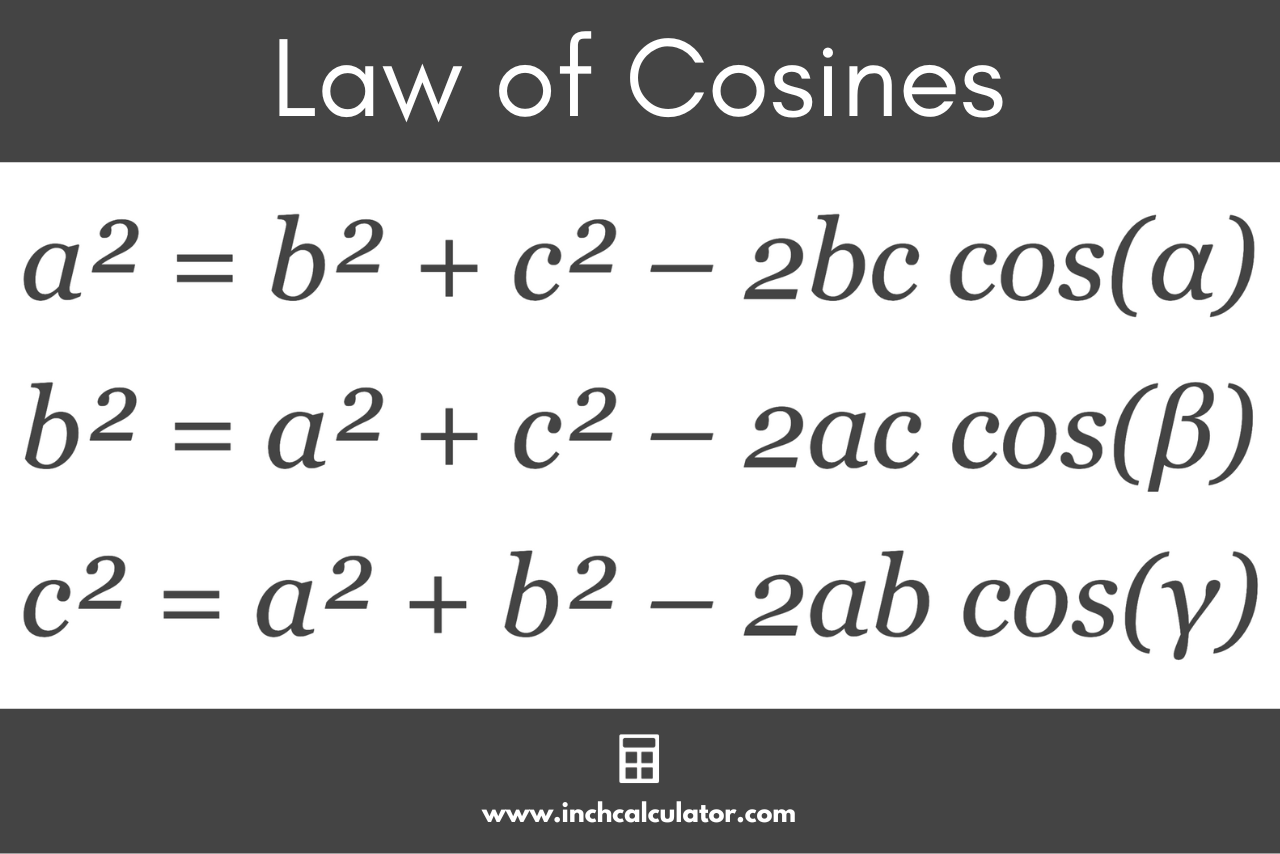If you know other properties of the triangle, you can solve the remaining sides and angles using the Law of Sines as well.

### How to Use the Law of Cosines to Solve Side Lengths

If you know the lengths of the other two sides of a triangle, you can use the Law of Cosines to determine the length of the remaining side. Rearranging the formulas, you can solve the length of any of the triangle’s sides as follows:

a = b² + c² – 2bc cos(α)
b = a² + c² – 2ac cos(β)
c = a² + b² – 2ab cos(γ)

This calculation is known as the side-angle-side theorem (SAS) and can be abstracted to solve any side length given the other two side lengths and the angle between them.

### How to Use the Law of Cosines to Solve Angles

You can also use the Law of Cosines to find the measure of an angle in a triangle. Rearranging the formulas, you can solve any of the angles in a triangle given the lengths of its sides as follows:

α = cos-1[b² + c² – a ² / 2bc]
β = cos-1[a² + c² – b ² / 2ac]
γ = cos-1[a² + b² – c ² / 2ab]

By using the inverse cosine of the right side of the equation, you can find the corresponding angle.

The resulting angle will be in radians, but you can convert it to degrees using our radians to degrees converter.

This calculation is known as the side-side-side theorem (SSS) and can be abstracted to solve any angle given the length of all three sides.

### Does the Law of Cosines apply to right triangles?

Yes! You can use the Law of Cosines to solve angles and side lengths for all types of triangles, including right triangles, equilateral triangles, and isosceles triangles.

### When can you use the Law of Cosines?

You can use the Law of Cosines to solve the angles of a triangle when you know the length of all three sides or to solve a triangle’s side length when you know the lengths of the remaining two sides and the angle between them.

### How does the Pythagorean theorem relate to the Law of Cosines?

Since the Law of Cosines is a generalization of the Pythagorean theorem, whenever you can use the Pythagorean theorem, you can also use the Law of Cosines. The Pythagorean theorem is a special case of the Law of Cosines such that when the cosine of an angle is equal to 0, the angle is a 90° right angle.

So, the Pythagorean theorem pertains to right triangles, while the Law of Cosines applies to all triangles, including those without a right angle.

## References

1. Michael Corral, Elementary Trigonometry - 2.2: The Law of Cosines, LibreTexts: Mathematics, https://math.libretexts.org/Bookshelves/Precalculus/Elementary_Trigonometry_(Corral)/02%3A_General_Triangles/2.02%3A_The_Law_of_Cosines